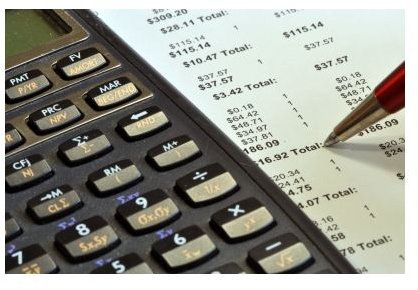# Different Forecasting Methods in Business

## Forecasting Sales, Inventory, Website Visitors, and MoreDifferent forecasting methods in business are available to improve decision-making and efficiencies. The type of forecasting method to use depends on the nature of the data and the level of accuracy the business is looking for. The most common business forecasting methods include regression analysis, moving average and Monte Carlo simulation.

## Regression Analysis

Regression analysis is a statistical forecasting model that predicts one variable based on its relationship with another variable. If two variables are deemed to have a strong relationship by calculating its coefficient, then a regression analysis can be used to make predictions. While it can be calculated by hand, programs such as Microsoft Excel can do the calculation easily. For instance, click on the “data” tab, select the “data analysis” button and then click on the “regression” option.

When calculating a regression analysis, the x variable and y variable must be determined. The x variable represents the data being used to make the prediction. For instance, if predicting the number of daily sales based number of daily website visitors, then the number of daily sales is the y variable and the website visitors is the x variable. If multiple variables need to be considered to make a prediction, then a multiple regression analysis should be done.

## Moving Average

The moving averages method of forecasting is especially useful for businesses wanting to make predictions in industries that are rapidly changing. Instead of looking at the average in a set period, the moving average looks at interval of time of which “moves” each week or month. A common approach of using this method is by taking the average of the previous three weeks to forecast the upcoming week. It can also be done for monthly or quarterly as well.

The main disadvantage of this method for forecasting is it does not take into consideration seasonal variations or upward demand trends. For instance, if the business is using a quarterly moving average and demand usually increasing significantly in the 4th quarter, then the moving average will be much too low. There is a technique called modified moving average (MMA), or the exponential moving average, in which resolves the shortcomings of the original moving averages method.

## Monte Carlo Simulation

The Monte Carlos simulation is a forecasting method that uses probabilities based on historical data, expertise or experience to make predictions. Businesses can create a range of possible values and the probability of each occurring, and then the simulation calculates what will most likely happen. The result of the model is recorded and typically repeated hundreds of times using different randomly selected variables.

Since the Monte Carlo simulation method relies on repeated computations, it is most efficient to use a computer instead of attempting this by hand. Computer software is available to calculate these simulations automatically. Microsoft Excel can also be used, but requires advanced formulas to set up the model. If the model is reliant on thousands of rows of data, it could Microsoft Excel may become slow or freeze.

## Using Different Forecasting Methods in Business

There are different forecasting methods in business for making certain types of predictions. If the business wants to make predictions of one variable based on another, a regression analysis could be used. For instance, to predict sales or advertisement revenue based on the number of website visitors, or the number of people that will attend a seminar based on how many members are in that city. A moving average is best used when it wants to make predictions based on the results of the recent past. This is most commonly used to predict the number of sales or when inventory should be replenished. The Monte Carlo simulation predicts what will happen in the future based on the probability of certain events occurring. This is typically used for managing risk or seeing how a decision will affect the company's performance. There are also common sales forecasting formulas that can be used in addition to these forecasting methods.